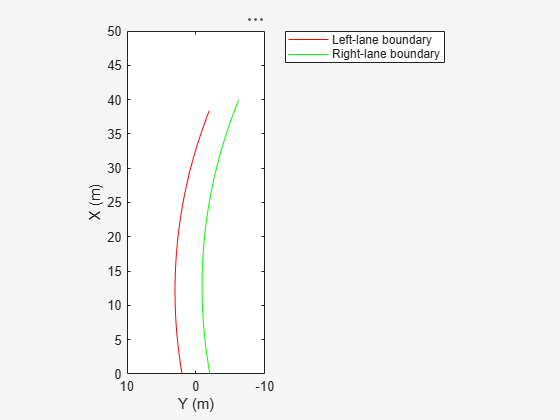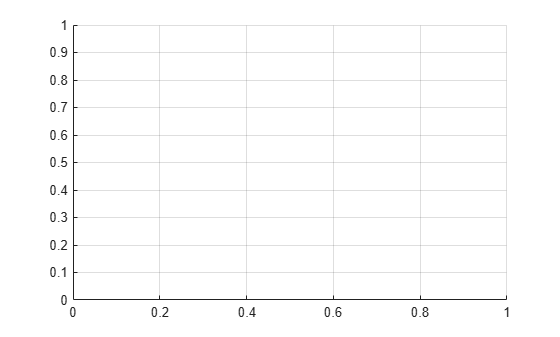Main Content

# computeBoundaryModel

Compute lane boundary points from clothoid lane boundary model

## Syntax

``yworld = computeBoundaryModel(boundary,xworld)``

## Description

``` `yworld = computeBoundaryModel(boundary,xworld)` returns the y-coordinates of lane boundary points, `yworld`, derived from a lane boundary, `boundary`, at points specified by the x-coordinates, `xworld`. All points are in world coordinates.```

## Examples

collapse all

Create clothoid curves to represent left and right lane boundaries. Then, plot the curves.

Create the left boundary.

```lb = clothoidLaneBoundary('BoundaryType','Solid', ... 'Strength',1,'Width',0.2,'CurveLength',40, ... 'Curvature',-0.8,'LateralOffset',2,'HeadingAngle',10);```

Create the right boundary with almost identical properties.

```rb = lb; rb.LateralOffset = -2;```

Create a bird's-eye plot. Then, create the lane boundary plotters and plot the boundaries.

```bep = birdsEyePlot('XLimits',[0 50],'YLimits',[-10 10]); lbPlotter = laneBoundaryPlotter(bep,'DisplayName','Left-lane boundary','Color','r'); rbPlotter = laneBoundaryPlotter(bep,'DisplayName','Right-lane boundary','Color','g'); plotLaneBoundary(lbPlotter,lb) plotLaneBoundary(rbPlotter,rb); grid hold on```Plot the coordinates of selected points along the boundaries.

```x = 0:5:50; yl = computeBoundaryModel(lb,x); yr = computeBoundaryModel(rb,x); plot(x,yl,'ro') plot(x,yr,'go') hold off```## Input Arguments

collapse all

Lane boundary model, specified as a `clothoidLaneBoundary` object.

x-world coordinates, specified as a real-valued vector of length N, where N is the number of coordinates.

Example: `2:2.5:100`

Data Types: `single` | `double`

## Output Arguments

collapse all

y-world coordinates, returned as a real-valued vector of length N, where N is the number of coordinates. The length and data type of `yWorld` are the same as for `xWorld`.

Data Types: `single` | `double`

## See Also

Introduced in R2018a

## Support

#### Implementing an Adaptive Cruise Controller with Simulink

Download technical paper